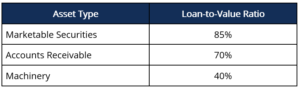# Asset-based Lending

A loan that is secured by an asset

## What is Asset-based Lending?

Asset-based lending refers to a loan that is secured by an asset. In other words, in asset-based lending, the loan granted by the lender is collateralized with an asset (or assets) of the borrower.### Quick Summary:

• Asset-based lending refers to a loan that is secured by an asset.
• Examples of assets that can be used to secure a loan include accounts receivable, inventory, marketable securities, and property, plant and equipment (PP&E).
• Lenders commonly use the loan-to-value ratio to determine the amount of money they are willing to lend.

### Understanding Asset-based Lending

In asset-based lending, the loan is secured by the assets of the borrower. Examples of assets that can be used to secure a loan include accounts receivable, inventory, marketable securities, and property, plant and equipment (PP&E). As the loan is secured by an asset, asset-based lending is considered less risky compared to unsecured lending (a loan that is not backed by an asset or assets) and, therefore, results in a lower interest rate charged. In addition, the more liquid the asset, the less risky the loan is considered and the lower the interest rate demanded.

For example, an asset-based loan secured by accounts receivable would be deemed safer than an asset-based loan secured by a property – the property is illiquid, and the creditor might find it difficult to liquidate the asset on the market quickly.

### Asset-based Lending Amount

Asset-based lending commonly references the loan-to-value ratio. For example, a lender may state “the loan-to-value ratio for this asset-based loan is 80% of marketable securities.” It states that the lender would only be willing to provide a loan of up to 80% of the value of the marketable securities. The loan-to-value ratio depends on the type of asset – lenders are generally willing to offer a higher loan-to-value ratio for more liquid assets. The loan-to-value ratio is calculated as follows:Where:

• Loan Amount is the amount that the lender is willing to loan; and
• Asset Value is the value of the asset being used as collateral for the loan.

Generally, the loan-to-value ratios for receivables and inventories are 70% and 50%, respectively.

### Example of Asset-Based Lending

A lender offers the following loan-to-value ratios for certain assets:A borrower requires a \$100,000 loan and owns the following assets:

• Marketable securities valued at \$105,000
• Accounts receivable valued at \$120,000
• Machinery valued at \$250,000

If the borrower is only able to use one asset to secure the loan, which asset should the borrower use to secure a loan of at least \$100,000?

• Marketable securities = \$105,000 x 85% = \$89,250 maximum loan amount;
• Accounts receivable = \$120,000 x 70% = \$84,000 maximum loan amount; and
• Machinery = \$250,000 x 40% = \$100,000 maximum loan amount.

The borrower should use machinery to secure the maximum loan.

Asset-based lending offers the following advantages to the borrower:

• Asset-based loans are easier and quicker to obtain than unsecured loans and lines of credit;
• Such loans generally include fewer covenants; and
• Asset-based loans generally come with a lower interest rate compared to other funding options.

Asset-based lending provides the following advantages for the lender:

• Asset-based loans are less risky as it is collateralized with an asset (or assets); and
• If the borrower defaults on the loan, the lender can obtain the assets that were used to secure the loan and liquidate them to settle the amount outstanding.